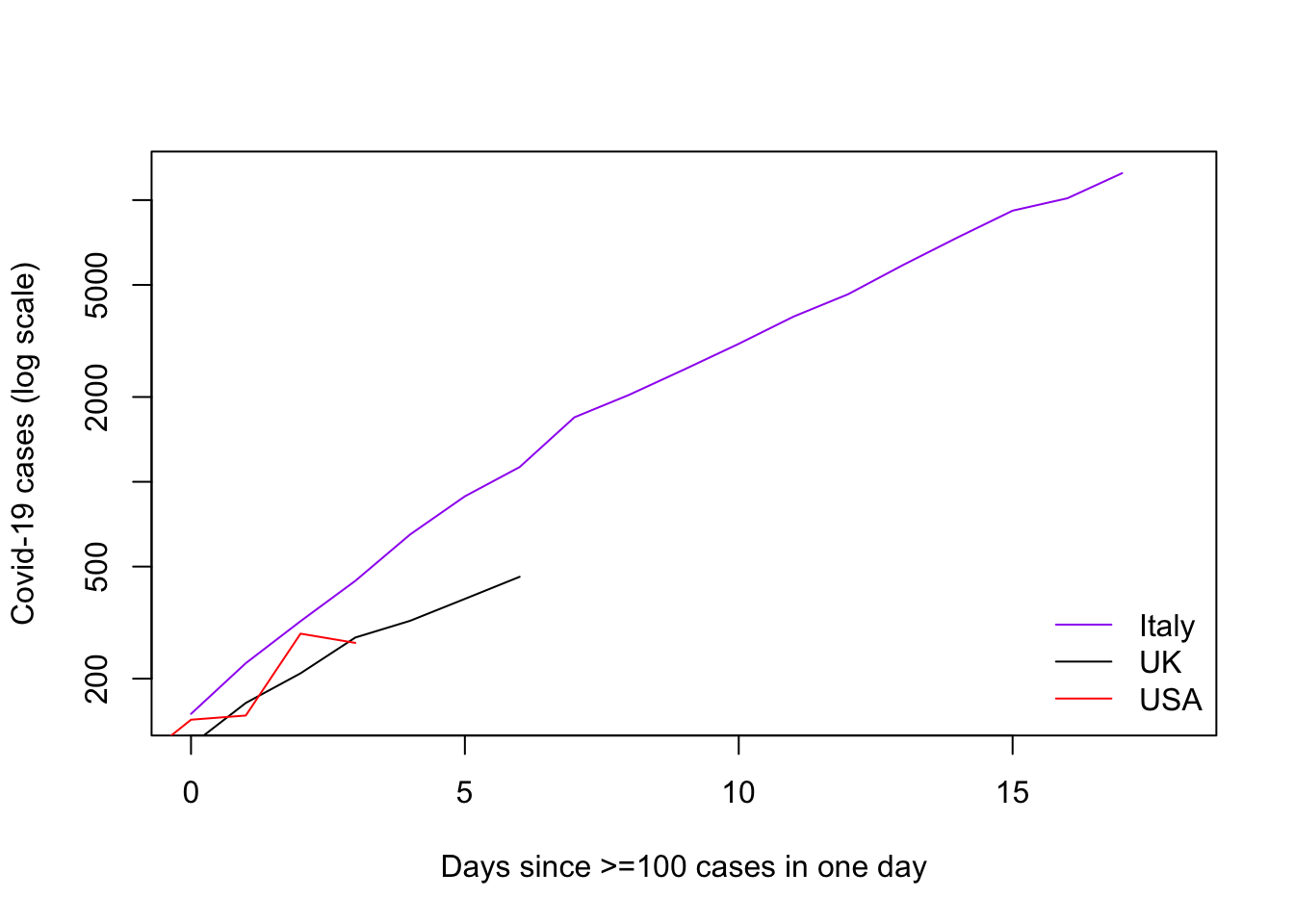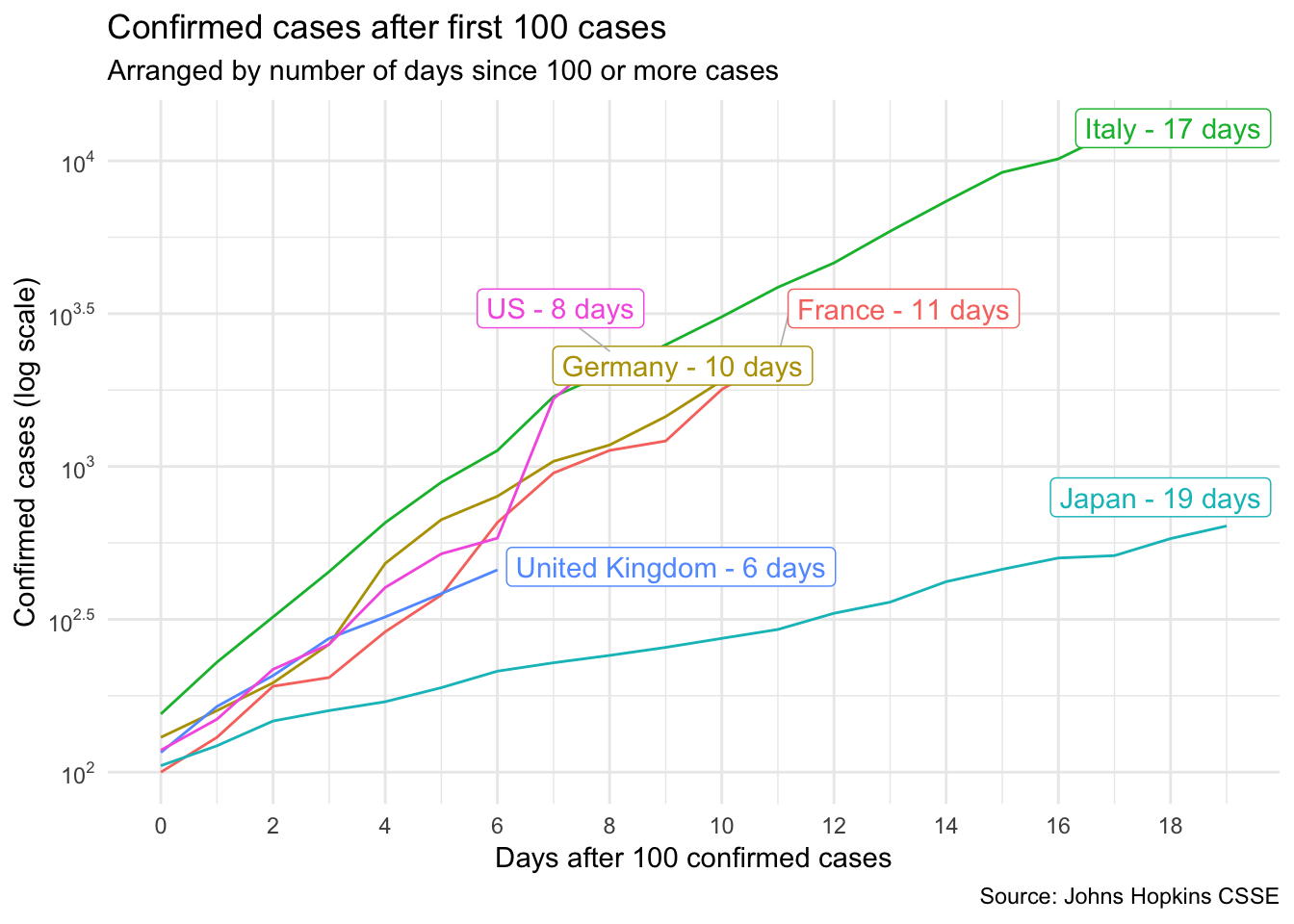I saw this graph on Twitter, and was asonished by the similarities in the growth rate of Covid-19 cases between countries. But I was frustrated that there was no code to explain how the graph was produced.

I tried to replicate the results by scraping data from Wikipedia (the code can be revealed if you wish).

I plotted the number of cases per day on the log scale, from the start of the epidemic in each country. I arbitrarity set the ‘start’ of tranmission in the country to the first day with 100 cases or more in one day. Because of this, Japan does not appear on this graph, despite being an important counterpoint in the linked graph. I also struggled to find easily accessible data for France and Spain, so have focused on Italy, UK, USA, and Germany.

# SCRAPING CODE FROM WIKIPEDIA
#=============================

# SET EPIDEMIC START VALUE
epi.cases <- 100

# ITALY
italy <- wiki_italy %>%
html_node(xpath = '//*[@id="mw-content-text"]/div/table') %>%
html_table(fill=TRUE) %>%
.[,c(1,24)] %>%
mutate(date = as.Date(Date, "%Y-%m-%d"),
cases = as.numeric(sub(',','',Confirmed))) %>%
filter(Date != 'Date' & !is.na(date)) %>%
select(date, cases)

# START OF THE EPIDEMIC IN ITALY
start <- italy$date[min(which(italy$cases>epi.cases))]

# UK
UK <- wiki_uk %>%
html_node(xpath = '//*[@id="mw-content-text"]/div/table') %>%
html_table(fill=TRUE) %>%
.[,c(1,14)] %>%
mutate(date = as.Date(Date, "%Y/%m/%d")) %>%
filter(!is.na(date))
UK$cases <- as.numeric(sub('','',sub('\\[.*','',UK[,2]))) UK <- UK[,c('date','cases')] # USA USA <- wiki_usa %>% html_node(xpath = '//*[@id="mw-content-text"]/div/table') %>% html_table(fill=TRUE) %>% .[,c(1,46)] %>% mutate(date = as.Date(Date, "%b %d")) %>% filter(!is.na(date)) USA$cases <- as.numeric(sub('','',sub('\\[.*','',USA[,2])))
USA <- USA[,c('date','cases')]

# GERMANY
germany <- wiki_germany %>%
html_node(xpath = '//*[@id="mw-content-text"]/div/table') %>%
html_table(fill=TRUE)
g <- rbind(germany, colnames(germany))
g <- g[c(1,20,21), !(colnames(g) %in% 'State')]
g <- as.data.frame(t(g))
g$date <- as.Date(paste0(g$21, g$1), '%B %d') g$cases <- as.numeric(as.character(sub('\\[.*','',g$20))) germany <- g[,c('cases', 'date')] # JAPAN -- not had more than 100 cases in a day # japan <- wiki_japan %>% # html_node(xpath = '//*[@id="mw-content-text"]/div/table') %>% # html_table(fill=TRUE) %>% # .[,c(1,2)] # japan$date <- as.Date(japan[,1], '%d %b %Y')
# japan$cases <- as.numeric(sub(',','',japan[,2])) The graph below does not replicate what was shown in the Tweet. Not sure why. Looks like UK might be on a different trajectory from Italy, and Germany have had some wobbles. # PLOTTING THE RESULTS #===================== plot(x = italy[italy$date>=start,'date'] - start,
y = italy[italy$date>=start,'cases'], xlim=c(0,Sys.Date()-start), log='y', xlab = 'Days since >=100 cases in one day', ylab = 'Covid-19 cases (log scale)', type='l', pch='.', col = 'purple') add.lines <- function(country, colour='black'){ lines(x = country$date - country$date[min(which(country$cases>epi.cases))],
y = country$cases, type='l', col = colour, pch='.') } add.lines(UK) add.lines(USA, 'red') #add.lines(germany, 'green') legend(x='bottomright', legend = c('Italy','UK','USA'), col=c('purple','black','red'), lty=1, bty='n')# Another graph using data from Hopkins USING CODE FROM: knitr::opts_chunk$set(echo = TRUE, warning = FALSE, message = FALSE)
# USING CODE FROM:
# https://github.com/JonMinton/COVID-19 # @JonMinton
# https://gist.github.com/christophsax/dec0a57bcbc9d7517b852dd44eb8b20b
# @christoph_sax
# https://github.com/gorkang/2020-corona/blob/master/2020-corona-plot.R#L18-L20

# Libraries ---------------------------------------------------------------

library(dplyr)
library(ggplot2)
library(ggrepel)
library(tidyr)
library(scales)

# Data prep ---------------------------------------------------------------

# Data Repo Johns Hopkins CSSE (https://github.com/CSSEGISandData/COVID-19)
url <- "https://raw.githubusercontent.com/CSSEGISandData/COVID-19/master/csse_covid_19_data/csse_covid_19_time_series/time_series_19-covid-Confirmed.csv"
dta_raw <- read_csv(url, col_types = cols()) %>% select(-Lat, -Long)

selection <- c("Italy",
#"Iran",
#"Spain",
#"South Korea",
"France",
"Germany",
"US",
"Japan",
#"Mainland China",
"United Kingdom")

dta <- dta_raw %>%

# tidy data
rename(province = Province/State, country = Country/Region) %>%
pivot_longer(c(-province, -country), "time") %>%
mutate(time = as.Date(time, "%m/%d/%y")) %>%

# rename some countries
mutate(
country = case_when(
country == "Iran (Islamic Republic of)" ~ "Iran",
country == "Hong Kong SAR"  ~ "Hong Kong",
country == "Republic of Korea" ~ "South Korea",
TRUE ~ country
)) %>%

# selection
filter(country %in% !! selection) %>%

# ignore provinces
group_by(country, time) %>%
summarize(value = sum(value)) %>%
ungroup() %>%

# calculate new infections
arrange(time) %>%
group_by(country) %>%
mutate(diff = value - lag(value)) %>%
ungroup() %>%
filter(!is.na(diff)) %>%
arrange(country, time)

DF_plot = dta %>%
filter(value >= 100) %>%
group_by(country) %>%
mutate(days_after_100 = 0:(length(country)-1)) %>%

# Create labels for last instance for each country
group_by(country) %>%
mutate(
name_end =
case_when(
value == max(value) ~ paste0(as.character(country), " - ", days_after_100, " days"),
TRUE ~ "")
)

# PLOT --------------------------------------------------------------------

plot1 = DF_plot %>%
ggplot(aes(x = days_after_100, y = value, color = country)) +
geom_line() +
ggrepel::geom_label_repel(aes(label = name_end), show.legend = FALSE, segment.color = "grey", segment.size  = .3) + #, segment.linetype = 5
scale_y_log10(
breaks = scales::trans_breaks("log10", function(x) 10^x),
labels = scales::trans_format("log10", scales::math_format(10^.x))) +
scale_x_continuous(breaks = seq(0, max(DF_plot\$value), 2)) +
labs(
title = "Confirmed cases after first 100 cases",
subtitle = "Arranged by number of days since 100 or more cases",
x = "Days after 100 confirmed cases",
y = "Confirmed cases (log scale)",
caption = "Source: Johns Hopkins CSSE"
) +
theme_minimal() +
theme(legend.position = "none")

plot1